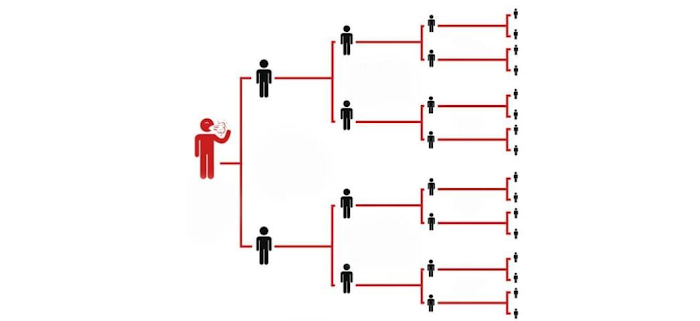# Did you about it part :- 2 UPSC CSC 2021 PRELIMS || BASIC REPRODUCTIVE RATIO||

Basic Reproductive Ratio

The basic reproductive ratio, pronounced as R-nought is defined.

await a number of second germs arising from a single.

the single during his or her entire virus period.

in a population of susceptible.

The larger the basic reproductive ratio the more contagious is the disease caused by the virus. It depends on the

number of days an infected person remains infective and the number

of susceptible persons available to infect This concept is fundamental to the study of epidemiology.

In biology what is the basic is the reproductive ratio?

It is like a fundamental concepts of mathematical ratio  biology defined the number of seconds infections caused  by a signal individual  R0

.

Rate of reproductive ratio?

The number is R0ALSO  called the ratio the incidence metric describes the infectious agents.

reproductive  of infection  is bodily fluids 1.5 – 2.5 in epidemiology called basic reproductive ratio or incorrectly  basic reproductive rate  and denoted R0, r naught cases generates on average over the course of its epidamic period in an otherhand  fresh crowd.

History of Ro

Originally developed for  demographics in 1886

And 1911 and 1927 is independently studied malaria  and in 1975+ now widely used for injections disease.

Heesterbeek & dietz 1996 says that one of the most valuable ideas that mathematical thinking has brought to the epidemic theory.

·       In it is individual lifetime they produced expected numbers of secondary individuals.

·       Secondary means lifetime demographics and ecology and number of individuals infected within a single infected individual entire infections lifetime epidemiology.

·       viruses cells produced by a single infected cell in host dynamics.

·       If there R0<1 then each individual produces on average less than one new  and hence the disease dies out

·       And if there is R>1 then each individual produces more than one new infected individuals on that time the disease is able to invade the susceptible population in our country.

·       Determines the effectiveness of control of measures.

·       R0 is a threshold we predict whether a disease will came endemic or die out

·       If R0 is R0>1  then each individual is causing more than one infection, so the disease will take hold of that

·       If R0 is the threshold and R<1  then the disease will die out  and most important in a threshold is where R0 is most useful

·       Ro is rarely measured in the field and Ro is an individual parameter, eg ODEs deal with populations matching the two and lots of thresholds , not necessarily the true Ro.

·       These surrogate, R0 like thresholds will also tell us whether a disease will become endemic or die out and they may be easier to calculate than the true R0 but it is different diseases.

·       Suppose HIV has an R0 of 2 and covid -19 has a Ro of 2.5, unless they were calculated using the same method, we do not know if covid 19 is worse than HIV  and we know that both will persist  because Ro is a distance measure and thus dependent on the metric used

·       Concepts of Anderson and May,

·       R0= b c d  where  B= transmission probability

·       C= number of contacts per unit time

·       D= average time spent infectious  is the method by  biologists not always correctly

·                                       THE JACOBIAN

·        A matrix of   partial derivatives, created by different every equation with respect to every variable and 6 equations and 6 variables and you will have  6 into 6 matrix

·        Calculate the disease of free equilibrium and the jacobian matrix after that evaluate the jacobian at the equilibrium and after that find the eigenvalues if all eigenvalues < 0 = stable even one eigenvalue >0 = unstable  and largest eigenvalue = r0 like threshold and use the real part.

·       R0 sis <1 we have the stability of the DFE and hence the disease dies out.

If R 0 sis >1 we have instability of the DFE and hence the disease persists but R 0 is still can not define.

{ Ro SIS }squre < 1 = dies out with diseases

[ Ro SIS ] square > 1 disease persists.

SIS model is threshold  sleight of hand Ro

There is no reason the largest eigenvalue would inexorably lead to the average number of infections

Ro is not defined  for ODEs, but we want to threshold then it will acceptable, there are so many methods of calculating that ratio.

SIS endemic equilibrium

The endemic equilibarium for the SIS model is b/a , n-b/a >0

N >b/a

Define R0 end = a N/b

As before [ since if  Ro end >1 , the disease persists

N = Total app

A= infection rate of sis

B=recovery rate

The gold standard of Ro
* complete cycle of account of dengue

Not restricted to ODEs but can get cucumber some for multiple states  just of particle use  we know scrapie in Cyprus ( Gravenor at 2004, dengue in brazil in 2003

The next generation of method

A general type of deriving Ro when the population is divided Into discrete, disjoint cases  will be  used as a model with underlying age people.

What is the mean of “generation”

The number of infected humans produced by a single infected human of all time  and next generation of infected mosquitos produced by a single human

In partical  : west nile virus { wonham 2004 } mad cow { 2003}

Recent world days

World FOOD safety day 2021; the theme, significance, history,

# World environment day 2021; learn how to celebrate this day of nature

Thanks for reading and watching this and do share more reach and give your valuable feedback 👉🏻👉🏻👉🏻👉🏻👉🏻 below